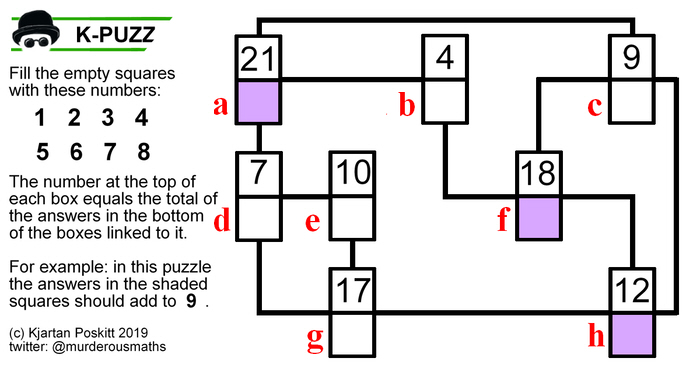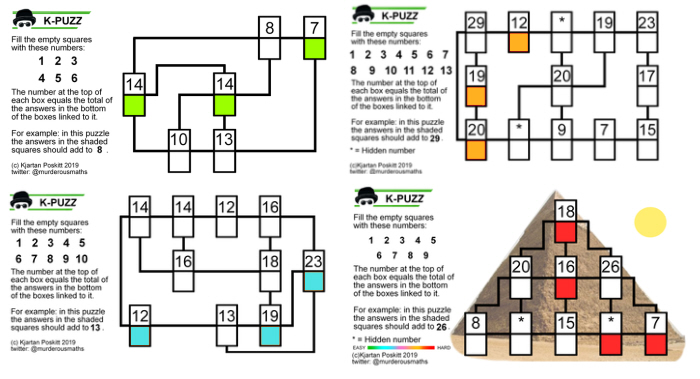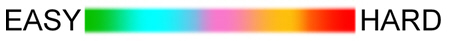# The K-PUZZ Logic PuzzlesK-PUZZ logic puzzles have appeared daily on the Murderous Maths Twitter Page since May 2019.
They are based on the original Kjarposko Puzzles which are usually larger and more complex.

This is how a typical K-PUZZ works. The red letters are for this explanation only. Numbers in [brackets] refer to the clues in the puzzle.In this puzzle, the answers a + f + h must add to . As a + f = , therefore h =  -  = 5 As b + c + h =  and we now know h = 5, therefore b + c =  - 5 = 13. Hold that thought. b + c + d = , but remember that b + c = 13, so d =  - 13 = 8 As d + g = , therefore g =  - 8 = 2 Let's see where we've got to...Another way to start this puzzle is to look at  and .  can only be made by adding 1 + 2 + 4 and  can only be made by adding 1 + 3. Therefore the 1 must go in the box which is common to both of them which is a. To complete , e = 4 and to complete , f = 3. To complete , c = 7 and finally b = 6. Here's the finished puzzle:## K-PUZZ variations

The puzzles can appear in a variety of shapes and formats, including novelty images.K-PUZZ can vary from EASY to HARD, indicated by the colour of the shaded squares. As a guide, the target time for an easy puzzle is 1 minute, and for a hard puzzle 5 minutes.

Some of the harder puzzles don't give all the clues, instead the numbers are replaced by an [*]